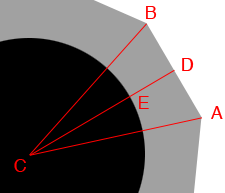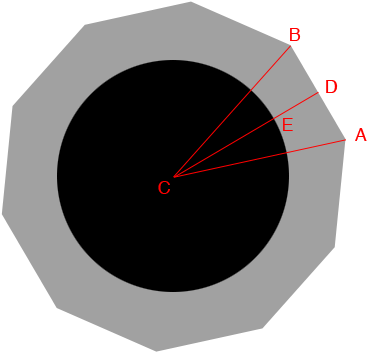SEARCH HOMEMath Central Quandaries & QueriesQuestion from Jayson, a parent: I have a round fire pit. It measures 25 inches in diameter. I have 12 inch long square bricks to go around it . My question is what degree do I cut the ends of these bricks to make them fit around this circle? The brick dimensions are 12"Lx6"Wx4"D.Hi Jayson,

I started by drawing one section of the fire pit with $C$ at the center of the pit and D the midpoint of the line section $AB.$The length of $CE$ is 12.5 inches and $ED$ is 6 inches and hence the length of $CD$ is 18.5 inches. $AD$ is 6 inches and hence

$\tan(DCA) = \frac{AD}{DC} = \frac{6}{18.5} = 0.3243$

and thus

$DCA = \tan^{-1}(0.3243) = 17.97 \mbox{ degrees.}$

and hence the measure of angle $BCA$ is $2 \times 17.97 = 35.93$ degrees which is very close to one tenth of 360 degrees and thus you will need 10 bricks.

Now i can draw the entire fire pit. The triangle $BCA$ is isosceles and hence the angles $ABC$ and $CAB$ have the same measure.The sum of the measures of the interior angles of a triangle is 180 degrees and hence the angle measures $CAB, ABC$ and $BCA$ add to 180. Since the measure of the angle $BAC$ is 36 degrees the measure of the angle $CAB$ is

$\frac12 (180 - 36) = 72 \mbox{ degrees.}$

I hope this helps,
HarleyMath Central is supported by the University of Regina and the Imperial Oil Foundation.